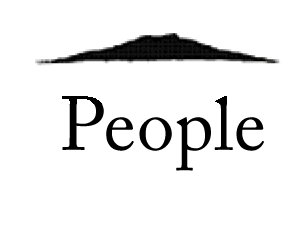#• Name：David HUGHES
• Position：1st year master's student in Mathematics
• Laboratory：Iwabuchi Laboratory
• Hometown：Dunboyne, County Meath, Ireland
• Favorite Books："Anarchy, State, and Utopia" by Robert Nozick
• Research area：Partial Differential Equations from Fluid Mechanics
• Posted Date：Jan 21, 2020

### １．What kind of the research are you doing?

In the context of fluid mechanics, partial differential equations are expressions of the speed and pressure of a given body of fluid within a given space, and how its speed and pressure change over time. When we use a mathematical equation to model a real physical phenomenon, we expect the equation to have certain properties. In particular, we would like a set of equations to be 'well posed'. This means that a unique solution to the equations exists, and that the solution changes continuously as we change the initial conditions of the equations.

My study is focused on these equations and attempting to prove that they satisfy these desirable properties.

### ２．What is the reason for starting your study?

I was inspired by Pierre Gilles Lemarie-Rieusset's book, "The Navier-Stokes Equations in the 21st Century". The Navier-Stokes equations are a set of equations describing the flow of fluids with a given level of viscosity. It is notoriously difficult to prove that they are well posed and behave like real physical fluid under general conditions. My undergraduate degree was in Mathematics and Theoretical Physics, so I have seen how useful these equations are in typical real-world situations.

This piqued my interest in the idea that the equations may or may not break down under more general settings, and so I decided to study them and other equations within a pure mathematical framework.

### ３．Message for prospective students

Tohoku University provides a great environment for devoting your time to study. You will make great progress in your learning, as well as great friends along the way.

TOPNext